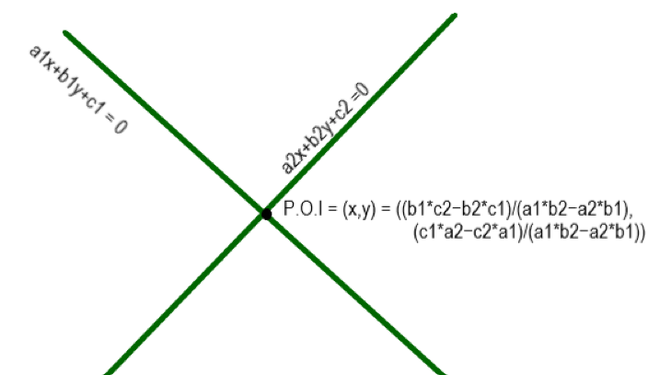Open In App
Related Articles
• Geometry in Maths

# Point of Intersection of Two Lines Formula

Point of intersection is the point where two lines or two curves meet each other. The point of intersection of two lines of two curves is a point. If two planes meet each other then the point of intersection is a line. More precisely it is defined as the common point of both the lines or curves that satisfy both the curves which can be derived by solving the equation of the curves.

If we consider two lines a1x + b1y + c1 = 0 and a2x + b2y + c2 = 0 the point of intersection of these two lines is given by:

Point of Intersection (x, y) = ((b1×c2 − b2×c1)/(a1×b2 − a2×b1), (c1×a2 − c2×a1)/(a1×b2 − a2×b1))Point of intersection

Derivation of point of intersection of two lines:

Given equations:

a1x + b1y + c1 = 0 -> eq-1

a2x + b2y + c2 = 0 -> eq-2

Solving the equations using cross multiplication method:

x     y     1

b1    c1    a1    b1

b2    c2    a2    b2

On cross-multiplying the constants we obtain:

x/(b1*c2 – b2* c1) = y/(c1*a2-c2*a1) = 1/(a1*b2-a2*b1)

Solving for x:

=> x/(b1*c2 – b2* c1) = 1/(a1*b2-a2*b1)

=> x = (b1*c2 – b2* c1)/(a1*b2-a2*b1)

Solving for y:

=> y/(c1*a2-c2*a1) = 1/(a1*b2-a2*b1)

=> y=(c1*a2−c2*a1)/(a1*b2−a2*b1)

Hence point of intersection:

(x,y) = ((b1×c2 − b2×c1)/(a1×b2 − a2×b1), (c1×a2 − c2×a1)/(a1×b2 − a2×b1))

If two lines are parallel they never intersect each other:

Condition for two lines a1x + b1y + c1 = 0, a2x + b2y + c2 = 0 to be parallel

a1/b1 = a2/b2.

### Sample Problems

Question 1: Find the point of intersection of line 3x + 4y + 5 = 0, 2x + 5y +7 = 0.

Solution:

The point of intersection of two lines is given by :

(x, y) = ((b1*c2−b2*c1)/(a1*b2−a2*b1), (c1*a2−c2*a1)/(a1*b2−a2*b1))

a1 = 3, b1 = 4, c1 = 5

a2 = 2, b2 = 5, c2 = 7

(x,y) = ((28-25)/(15-8), (10-21)/(15-8))

(x,y) = (3/7,-11/7)

Question 2: Find the point of intersection of line 9x + 3y + 3 = 0, 4x + 5y + 6 = 0.

Solution:

The point of intersection of two lines is given by :

(x,y) = ((b1*c2−b2*c1)/(a1*b2−a2*b1), (c1*a2−c2*a1)/(a1*b2−a2*b1))

a1 = 9, b1 = 3, c1 = 3

a2 = 4, b2 = 5, c2 = 6

(x, y) = ((18-15)/(45-15), (54-12)/(45-15))

(x, y) = (1/10, 7/5)

Question 3: Check if the two lines are parallel or not  2x + 4y + 6 = 0, 4x + 8y + 6 = 0

Solution:

To check whether the lines are parallel or not we need to check a1/b1 = a2/b2

a1 = 2, b1 = 4

a2 = 4, b2 = 8

2/4 = 4/8

1/2 = 1/2

Since the condition is satisfied the lines are parallel and can’t intersect each other.

Question 4: Check if the two lines are parallel or not  3x + 4y + 8 = 0, 4x + 8y + 6 = 0

Solution:

To check whether the lines are parallel or not we need to check a1/b1 = a2/b2

a1 = 3, b1 = 4

a2 = 4, b2 = 8

3/4 is not equal to 4/8

Since the condition is not satisfied the lines are not parallel.

Question 5: Check whether the point (3, 5) is point of intersection of lines 2x + 3y – 21 = 0, x + 2y – 13 = 0.

Solution:

A point to be a point of intersection it should satisfy both the lines.

Substituting (x,y) = (3,5) in both the lines

Check for equation 1: 2*3 + 3*5 – 21 =0 —-> satisfied

Check for equation 2: 3 + 2* 5 -13 =0 —-> satisfied

Since both the equations are satisfied it is a point of intersection of both the lines.

Question 6: Check whether the point (2, 5) is point of intersection of lines x + 3y – 17 = 0, x + y – 13 = 0

Solution:

A point to be a point of intersection it should satisfy both the lines.

Substituting (x,y) = (2,5) in both the lines

Check for equation 1: 2+ 3*5 – 17 =0 —-> satisfied

Check for equation 2: 7 -13 = -6  —>not satisfied

Since both the equations are not satisfied it is not a point of intersection of both the lines.

Question 7: Find the point of intersection of lines x = -2 and 3x + y + 4 = 0

Solution:

On substituting x = -2 in 3x + y + 4 = 0

-6 + y + 4 = 0;

y = 2;

So the point of intersection is (x,y) = (-2,2)